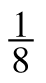### Home > CCA > Chapter 8 > Lesson 8.1.2 > Problem8-19

8-19.

Write the equation for the following two sequences in “first term” form. Homework Help ✎

1. $\frac { 1 } { 2 }$, $\frac { 1 } { 4 }$,, ...

Look at the first number in the sequence and determine how much each following term increases or decreases.

$\text{In this case, }\frac{1}{2} \text{ is the first term.}$

$\text{The terms decrease by a factor of }\frac{1}{2}.$

$\textit{t}(\textit{n})=\frac{1}{2}\left(\frac{1}{2}\right)^{\textit{n}-1}$

1. $-7.5,-9.5,-11.5,\dots$

Note that each term decreases by $2$.